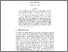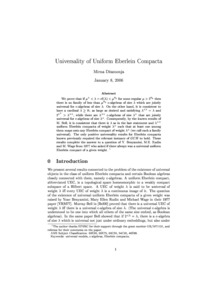# Universality of uniform Eberlein compacta

Džamonja, M (2006) Universality of uniform Eberlein compacta. Proceedings of the American Mathematical Society, 134 (8). pp. 2427-2435.Preview Postscript (unifEber2.ps) Download (208kB) | Preview

## Abstract

We prove that if $\mu^+ 2^{\aleph_0}$, then there is no family of less than $\mu^{\aleph_0}$ c-algebras of size $\lambda$ which are jointly universal for c-algebras of size $\lambda$. On the other hand, it is consistent to have a cardinal $\lambda\ge \aleph_1$ as large as desired and satisfying $\lambda^{\lambda^{++}$, while there are $\lambda^{++}$ c-algebras of size $\lambda^+$ that are jointly universal for c-algebras of size $\lambda^+$. Consequently, by the known results of M. Bell, it is consistent that there is $\lambda$ as in the last statement and $\lambda^{++}$ uniform Eberlein compacta of weight $\lambda^+$ such that at least one among them maps onto any Eberlein compact of weight $\lambda^+$ (we call such a family universal). The only positive universality results for Eberlein compacta known previously required the relevant instance of $GCH$ to hold. These results complete the answer to a question of Y. Benyamini, M. E. Rudin and M. Wage from 1977 who asked if there always was a universal uniform Eberlein compact of a given weight.

Item Type: Article Faculty of Science > School of Mathematics Faculty of Science > Research Groups > Logic Vishal Gautam 18 Mar 2011 10:19 15 Dec 2022 18:30 https://ueaeprints.uea.ac.uk/id/eprint/19957 10.1090/S0002-9939-06-08189-5View Item